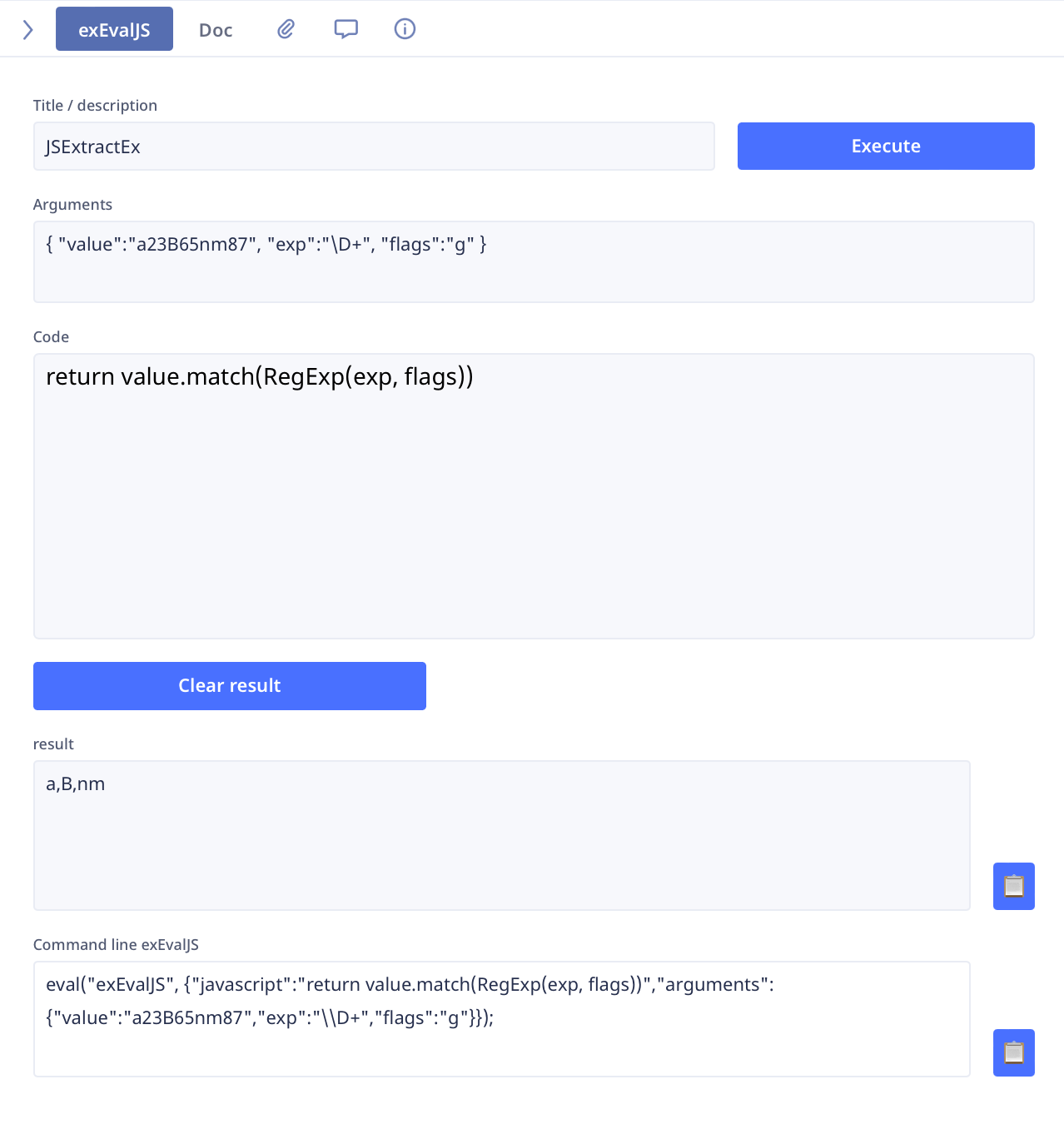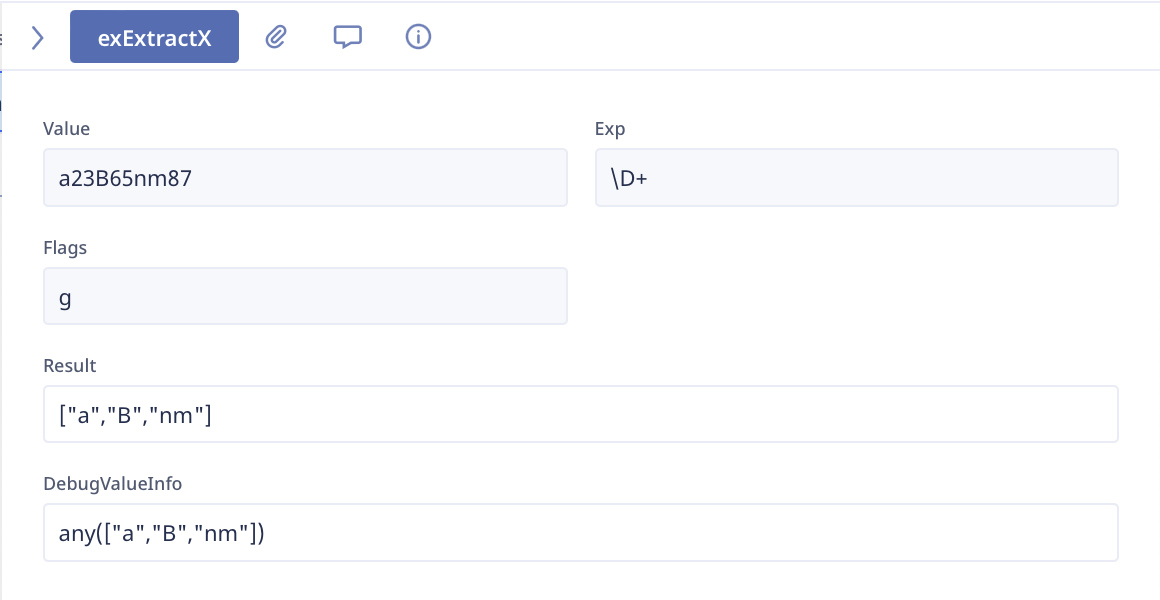1# extractx() “g” flag workaround

Here is a basic UDF for extracting all of the values that match a regex pattern...

`function extractxg(src : text,regex : text) do`
`let extValue := “”;`
`let idx := 0;`
`let idxLen := 0;`
`while testx(src, regex) do `
`idx := index(src, extractx(src, regex));`
`idxLen := length(extractx(substr(src, idx), regex));`
`extValue := extValue + extractx(substr(src, idx), regex) + “,”;`
`src := substr(src, idx + idxLen)`
`end`
`;`
`if extValue = “” then`
`extValue := null`
`else`
`extValue := substr(extValue, 0, length(extValue) - 1)`
`end;`
`extValue`
`end`

Using this example...

`let myVar := “a23B65nm87”;`
`extractxg(myVar, “\D+”)`

a,B,nm is returned as a concatenated string.

13replies Oldest first
• Oldest first
• Popular•• Sean
• Sean
• 7 mths ago
• Reported - view

Just updated versions. I prefixed function names with "gfn" to describe global functions. As the names imply, "NoFlags" does not allow flags and "Flags" requires flags.

`function gfnExtractxGNoFlags(src : text,regex : text,grp : text) do`
` let extractedValue := "";`
` let idx := 0;`
` let idxLen := 0;`
` while testx(src, regex) do `
` idx := index(src, extractx(src, regex));`
` idxLen := length(extractx(substr(src, idx), regex));`
` extractedValue := extractedValue + if grp = "" then`
` if extractx(substr(src, idx), regex) != null then`
` extractx(substr(src, idx), regex) + "¶"`
` end`
` else`
` if extractx(substr(src, idx), regex, grp) != null then`
` extractx(substr(src, idx), regex, grp) + "¶"`
` end`
` end;`
` src := substr(src, idx + idxLen)`
` end`
`;`
` if extractedValue != "" then`
` extractedValue := substr(extractedValue, 0, length(extractedValue) - 1)`
` end;`
` if extractedValue != "" then`
` split(extractedValue, "¶")`
` else`
` null`
` end`
`end;`

`function gfnValidateFlags(flags : text) do`
` let validFlags := "";`
` if length(flags) != 0 then`
` for i in range(0, length(flags)) do`
` switch item(flags, i) do`
` case "i":`
` (validFlags := validFlags + "i")`
` case "m":`
` (validFlags := validFlags + "m")`
` case "s":`
` (validFlags := validFlags + "s")`
` case "u":`
` (validFlags := validFlags + "u")`
` end`
` end`
` end;`
` validFlags`
`end;`

`function gfnExtractxGFlags(src : text,regex : text,flags : text,grp : text) do`
` let extractedValue := "";`
` let validFlags := gfnValidateFlags(flags);`
` let idx := 0;`
` let idxLen := 0;`
` while testx(src, regex, validFlags) do `
` idx := index(src, extractx(src, regex, validFlags, "\$0"));`
` idxLen := length(extractx(substr(src, idx), regex, validFlags, "\$0"));`
` extractedValue := extractedValue + if extractx(substr(src, idx), regex, validFlags, grp) != null then`
` extractx(substr(src, idx), regex, validFlags, grp) + "¶"`
` end;`
` src := substr(src, idx + idxLen)`
` end`
`;`
` if extractedValue != "" then`
` extractedValue := substr(extractedValue, 0, length(extractedValue) - 1)`
` end;`
` if extractedValue != "" then`
` split(extractedValue, "¶")`
` else`
` null`
` end`
`end`

Like
•• Sean
• Sean
• 2 mths ago
• Reported - view

I was inspired by Jacques TUR work with JavaScript in Ninox and decided to try and make the extractx() function work by modifying the code in the main.js file in the Applications/Ninox Database folder. I know this will appeal only to a very small number of users and maybe only me, but I wanted to share. And, who knows, maybe one of the Ninox developers will actually fix it eventually. The splitx() and replacex() functions have their uses, but I think the extractx() function is very powerful and is underserved by not implementing the "g" or global flag. I found out how to implement the global flag here...

and here

Here is the original function code...

``````Hr("extractx(string,string,string,string)", gl.tstring, (function(e, t, i, o) {
if (null == e)
return "";
if (null == t)
return "" + e;
null == i && (i = ""),
null == o && (o = "");
try {
var a = new RegExp(t, i).exec(e);
if (a) {
for (;;) {
var n = /\\$([0-9]*)/.exec(o);
if (!n)
break;
o = o.substr(0, n.index) + a[parseInt(n, 10)] + o.substr(n.index + n.length)
}
return o
}
return
} catch (e) {
return "#ERR: " + String(e)
}
}))``````

Here is the code I replaced it with...

``````Hr("extractx(string,string,string,string)", gl.tstring, (function(e, t, i, o) {
if (null == e)
return "";
if (null == t)
return "" + e;
null == i && (i = ""),
null == o && (o = "");
try {
let a = new RegExp(t, i).exec(e);
let regex = new RegExp(t, i);
let match;
let og;
let n;
let result = [];
if (a) {
if (i.includes('g')) {
while ((match = regex.exec(e)) && match !== '') {
og = o;
while ((n = /\\$([0-9]*)/.exec(og)))
og = og.substr(0, n.index) + match[parseInt(n, 10)] + og.substr(n.index + n.length);
result.push(og);
}
} else {
while ((n = /\\$([0-9]*)/.exec(o)) !== null)
o = o.substr(0, n.index) + a[parseInt(n, 10)] + o.substr(n.index + n.length);
result.push(o);
}
return result;
}
return
} catch (e) {
return "#ERR: " + String(e)
}
}))
``````

This is not helpful for the browser version, but for anyone who is willing to modify their local main.js file it works... until Ninox updates the app and then you have to do it again. Btw, the Ninox main.js file has no white-space so I have a no white-space version for anyone who doesn't want to mess with removing it.

Like
•Brilliant Sean!!!That transports me to another topic, the report print engine which doesn't handle HTML. On the web version the engine is not local, it's on the Ninox server, but on the Mac app it must be local and I may be able to find out why it's not working and find a solution.

Like 1
•For cloud users (and for those on mac and ipad), you can also use the extended functions and call the regular expressions functions directly from JavaScript.

I make an example in the demo application which is on GitHub ( it uses the extended functions facility, see on main page on GitHub and on related topic about JavaScrip call)In the end, the Ninox code to use is as follows:

``````eval("exEvalJS", {
"javascript":"return value.match(RegExp(exp, flags))",
"arguments":{
"value":"a23B65nm87",
"exp":"\\D+",
"flags":"g"
}
});``````

For ease of use, you can also encapsulate it in a global function by giving it the same name as the Ninox function: extractx.

``````function extractx(value : text,exp : text,flags : text) do
eval("exEvalJS", {
javascript: "return value.match(RegExp(exp, flags))",
arguments: {
value: value,
exp: exp,
flags: flags
}
})
end``````

so you can use the new function as if Ninox updated its function. this saves you from having to modify Ninox (although I love this idea)

``var result := extractx("a23B65nm87", "\D+", "g" );``

I also had fun making a form to test the global function:Many thanks Sean for re-launching this topic, as regular expressions are powerful tools for string management which are very useful to manage number formats (phone number, text and number separation...).

I use this website a lot to define and test regular expressions : https://regex101.com/r/cO8lqs/3

Like 1
•• Mike
• ninox.1
• 2 mths ago
• Reported - view

I use RegExes all the time also, but being new to Ninox, where do you put your "UDF" and how do you trigger it. I'm so new to Ninox, I still am not able to update a field from another field...

Like
•• Sean
• Sean
• 2 mths ago
• Reported - view

I readily admit I'm not the best communicator and I have a tendency to underexplain rather than overexplain a point. I figure if someone needs clarification they will ask.

This is something that is not in the documentation, but is required to make the 4 parameter version of extractx() work and this includes the user-defined functions. The last parameter, groups, must have a value for the function to return anything.

• If you don't want specific capture groups, use "\$0" in the 4th parameter
• if you want capture groups, use "\$1" up to "\$9" and you can include separating text if you like, e.g., "\$1 is a member of \$2"

I know I have seen "\$0" referred to on the forum, but this is the only thread I can find at the moment... https://forum.ninox.com/t/g9hrm3g#83hrraf. I have also seen it on the German language forum.

Here is the link to the extractx() function documentation... https://docs.ninox.com/en/script/functions/extractx. There are literally 3 separate functions in the main.js file, one for each of functions listed in the documentation.